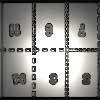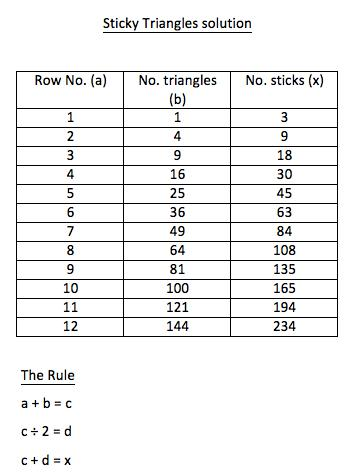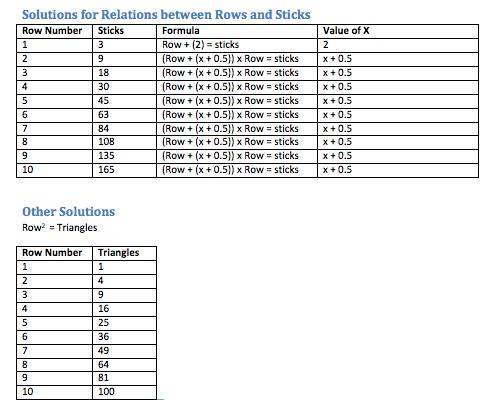#### You may also like### Exploring Wild & Wonderful Number Patterns

EWWNP means Exploring Wild and Wonderful Number Patterns Created by Yourself! Investigate what happens if we create number patterns using some simple rules.### Magazines

Let's suppose that you are going to have a magazine which has 16 pages of A5 size. Can you find some different ways to make these pages? Investigate the pattern for each if you number the pages.### Pebbles

Place four pebbles on the sand in the form of a square. Keep adding as few pebbles as necessary to double the area. How many extra pebbles are added each time?

# Sticky Triangles

##### Age 7 to 11 Challenge Level:

We had a large number of solutions and ideas sent in for this activity.

St. Mary MacKillop Primary school in Australia sent the following:

Triangles  Matchsticks
1                3
4                9
9               18
16               30
25               45

We found lots of patterns in both the triangles and matchsticks. The matchstick columns were all multiples of 3 and each new line added created a total of another multiple of 3. The number of triangles were increasing in odd numbers or adding two each row.

From Brookfield Junior School we had solutions from Lewis, Emma, Jodie, Maddie, Leah, Luke Alisha, Ronnie and here is Bill's:

To find out the amount of triangles in each pattern, you would do T=N$^2$ so if it was pattern twelve you will have 144 triangles because you would do 12$^2$ which is 144.
To find out the amount of matches if it was the ninth pattern you would add all the numbers up to nine and times it by three because there are 3 matches in a triangle. So for pattern 12, you would do 1+2+3+4+5+6+7+8+9=78 so then 78x3=234 so in pattern 12 there would be 234 matches this is the formula to sticky triangles.

Jenny, Ly, Phan Ha and Joseph at the  British International School, HCMC Vietnam said:

1 T 3 sticks 3 x 1
4 T  9 sticks 3 x 3
9 T 18 sticks 3 x 6
16 T 30 sticks 3 x 10
25 T 45 sticks 3 x 15
36 T 63 sticks 3 x 21
49 T 84 sticks 3 x 28
64 T 108 sticks 3 x 36

T:Triangle

First we started by counting the sticks and the triangles. We soon noticed that:
1 T 3 sticks 3 x 1
4 T  9 sticks 3 x 3
9 T 18 sticks 3 x 6

First column (Ts):
Like we said earlier, we started by counting the triangles. When we reached 16 T we noticed that the number of triangles increase by consecutive square numbers. So we stopped counting and used the pattern we noticed.

Sticks:
Again, we started by counting the sticks. Then we soon noticed a pattern that led us to a third column. After reaching up to 16 T we spotted a pattern in the third column.

1 T 3 sticks 3*1
4 T  9 sticks 3*3
9 T 18 sticks 3*6
16 T 30 sticks 3*10

How did we continue to add sticks without counting:
First we figured out the numbers that we have to times to get the amount of sticks (x3).Then we found another pattern - the differences:
The difference between 1 and 3=2
The difference between 3 and 6=3
The difference between 6 and 10=4
And the pattern continues by consecutive numbers.

Tristan from Lamerton C of E Primary Schoold wrote:

1. I made a triangle of four layers of triangles.
2. I recorded some data including:
- number of matchsticks in my four-layer triangle
- the upright triangles
- the upside down triangles
- the total number of triangles.
I repeated this process on a three-layer triangle, a two-layer and a one-layer.
3. I looked for patterns such as the total triangles are triangular numbers but the most important pattern for working out the formula was that the number of matchsticks was three times more than the number of upright triangles.
4 This led me to creating an expression n= number of layers, or if you were to lay the triangles out in height order, it would be the nth term of that sequence. The expression looked like this.
3 (n(n+1))= number of sticks

Taylor from Canberra Grammar School in Australia sent in a document which can be viewed here Taylor .doc

From Martha, Max, Felix, Charlie and Matthe at St Mary's Primary School Chipping Norton we had a good selection of ideas including;Well done all of those who sent in solutions. You worked well on this activity.
The following solution came in at the end of July 2014 from Mustafa at Redlands Primary School in Reading.